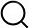# Also in the Article

Hertz contact model with surface tension
This protocol is extracted from research article:
The surface stress of biomedical silicones is a stimulant of cellular response

Procedure

The contact problem is shown in fig. S6D. A rigid sphere with radius R indents on a half space by its own weight. A buoyance-corrected gravitational force F is applied on the substrate along the z dimension, resulting in an indentation depth d and a circular contact area with radius a.

The solution of this contact problem can be obtained using a recent work by Hui et al. (28). Let a* and d* denote the predicted contact radius and indentation depth for the classical Hertz model, which does not account for surface tension. For an incompressible elastic substrate with Young’s modulus E, the apparent hardness F/(a*d*) predicted by Hertz theory is$Fa*d*=16E9$(1)

The result of Hui et al. predicts that the hardness, corrected for surface tension, is$Fad=16E9PH¯(32ω)$(2)where $PH¯(32ω)$ is the dimensionless function$PH¯(32ω)=1+9π8ω[(32ω)2+0.6016(32ω)+0.0171(32ω)2+0.3705(32ω)+0.0063]$(3)which depends only on the elastocapillary number$ω=a∙Eγ$(4)where γ is the surface tension. The function $PH¯(32ω)$ is a correction term that compensates for surface tension. For example, for small elastocapillary number, $32ω→0$, surface tension effect becomes insignificant; $PH¯(32ω)$ goes to one, and a*d* = ad. Specifically, the ratio of the observed and Hertz or apparent hardness is obtained by dividing Eq. 2 by Eq. 1. This results in$a*d*ad=PH¯(32ω)$(5)

The apparent hardness given by Eq. 1 can be computed using the buoyance-corrected gravitational force and the modulus of substrate. Using Eq. 5 above, fig. S6D plots the ratio of observed to predicted hardness, a*d*/ad, versus the elastocapillary number, a·E/γ.

Note: The content above has been extracted from a research article, so it may not display correctly.

Q&A